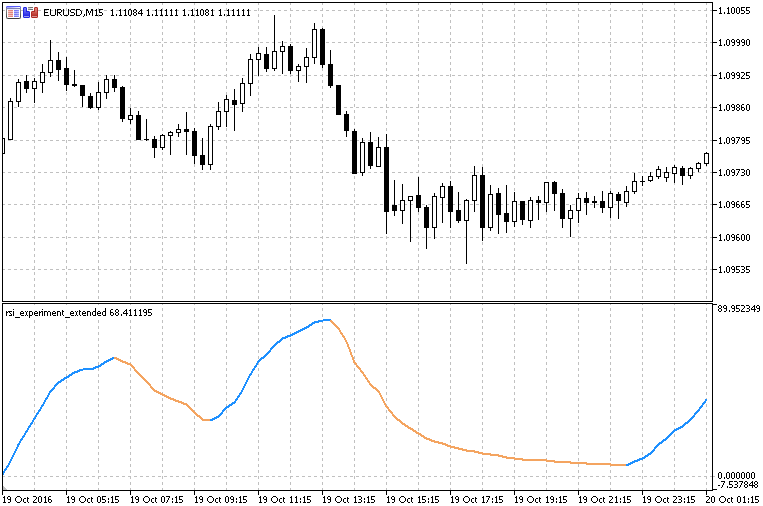Interesting script?
So post a link to it -
let others appraise it

You liked the script? Try it in the MetaTrader 5 terminal# RSI Experiment - indicator for MetaTrader 5

Views:
4891
Rating:
Published:
2016.11.04 15:35

This version of RSI is "experimenting" with different ways how internally the RSI is calculated. Welles Wilder used what is frequentlyy called "Wilders EMA" which is, in fact, exactly the same as smoothe MA (SMMA).

So, in this version, we can chose which one of the 4 basic averages can be used for RSI calculation, and that way we can get 3 completely new types odf RSI.

Additionaly, price can be smoothed prior to RSI calculation in which case we are getting what is usually regered to as RSI of MA (rsioma) and even then we are getting completely new versions of RSI of MA. With this "experiment" — with a simple average type change in the calculation we are having completely new types of rsi / rsioma.T3 Velocity on Chart

T3 velocity, now on chart version too.T3 Velocity V.2.0

T3 velocity is a completely new indicator that uses T3 to calculate velocity.RSI Experiment with Floating Levels

RSI experiment with floating levels.RSI Exp with Filled Areas

RSI experiment with a simple addition of filled areas when floating levels are crossed.